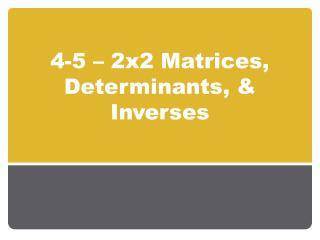DownloadDownload Presentation4-5 – 2x2 Matrices, Determinants, & Inverses

# 4-5 – 2x2 Matrices, Determinants, & Inverses

Download Presentation## 4-5 – 2x2 Matrices, Determinants, & Inverses

- - - - - - - - - - - - - - - - - - - - - - - - - - - E N D - - - - - - - - - - - - - - - - - - - - - - - - - - -
##### Presentation Transcript

1. 4-5 – 2x2 Matrices, Determinants, & Inverses

2. Objectives Evaluating Determinants of 2x2 Matrices Using Inverse Matrices to Solve Equations

3. Vocabulary A square matrix is a matrix with the same number of columns as rows. Multiplicative Identity Matrix is an n x n square matrix with 1’s along the main diagonal and 0’s elsewhere.

4. Vocabulary Multiplicative Inverse of a Matrix If A and B are n x n matrices, and AB = BA = 1, then B is the multiplicative inverse of A, written A -1 -1 -1 AA = A A = 1

5. 3 –1 7 1 0.1 0.1 –0.7 0.3 AB = (3)(0.1) + (–1)(–0.7) (3)(0.1) + (–1)(0.3) (7)(0.1) + (1)(–0.7) (7)(0.1) + (1)(0.3) = 1 0 0 1 = Verifying Inverses Show that matrices A and B are multiplicative inverses. 3 –1 7 1 0.1 0.1 –0.7 0.3 A = B = AB = I, so B is themultiplicative inverse of A.

6. Vocabulary The determinant of a 2 x 2 matrix is ad – bc. det A

7. 78 –5–9 = = (7)(–9) – (8)(–5) = –23 4–3 56 = = (4)(6) – (–3)(5) = 39 a–b ba = = (a)(a) – (–b)(b) = a2 + b2 Evaluating the Determinant of a 2x2 Matrix Evaluate each determinant. 7 8 –5 –9 a. det b. det c. det 4 –3 5 6 a –b ba

8. Vocabulary Let A = . If det A = 0, then A has no inverse. If det A ≠ 0, then A =

9. = / 12 4 9 3 6 5 25 20 Since the determinant 0, the inverse of Y exists. Finding an Inverse Matrix Determine whether each matrix has an inverse. If it does, find it. a.X = Find det X. ad – bc = (12)(3) – (4)(9) Simplify. = 0 Since det X = 0, the inverse of X does not exist. b.Y = Find det Y. ad – bc = (6)(20) – (5)(25) Simplify. = –5

10. 20–5 Change signs. –256 Switch positions. 1 det Y 20 –5 Use the determinant to –25 6 write the inverse. 1 det Y = 20 –5 Substitute –5 for the –25 6 determinant. 1 5 = – –4 1 5 –1.2 = Multiply. Continued (continued) Y–1 =

11. d–b –ca Use the definition of inverse. 1 ad – bc A–1 = 11–25 –49 1 (9)(11) – (25)(4) Substitute. = –11 25 4 –9 Simplify. = –11 25 4 –9 3 –7 Substitute. X = Solving a Matrix Equation 9 25 4 11 3 –7 Solve X = for the matrix X. The matrix equation has the form AX = B. First find A–1. Use the equation X = A–1B.

12. Multiply and simplify. (–11)(3) + (25)(–7) (4)(3) + (–9)(–7) –208 75 = = Check: Use the original equation. 9 25 4 11 3 –7 X = 9 25 4 11 –208 75 3 –7 Substitute. 9(–208) + 25(75) 4(–208) + 11(75) 3 –7 Multiply and simplify. 3 –7 3 –7 = Continued (continued)

13. From AB To A To B 0.84 0.07 0.16 0.93 Write the percents as decimals. Real World Example In a city with a stable group of 45,000 households, 25,000 households use long distance carrier A, and 20,000 use long distance carrier B. Records show that over a 1-year period, 84% of the households remain with carrier A, while 16% switch to B. 93% of the households using B stay with B, while 7% switch to A. a. Write a matrix to represent the changes in long distance carriers.

14. Use A Use B 25000 20000 Write the information in a matrix. 0.84 0.07 0.16 0.93 25000 20000 22,400 22,600 = Continued (continued) b. Predict the number of households that will be using distance carrier B next year. 22,600 households will use carrier B.

15. 0.84 0.07 0.16 0.93 First find the determinant of . 0.84 0.07 0.16 0.93 = 0.77 1 0.77 25,000 20,000 28,377 16,623 Multiply the inverse matrix by the information matrix in part (b).Use a calculator and the exact inverse. 0.93 –0.07 –.016 0.84 Continued (continued) c. Use the inverse of the matrix from part (a) to find, to the nearest hundred households, the number of households that used carrier A last year. About 28,400 households used carrier A.

16. Homework Pg 203 # 1,4,5,14,18, 22